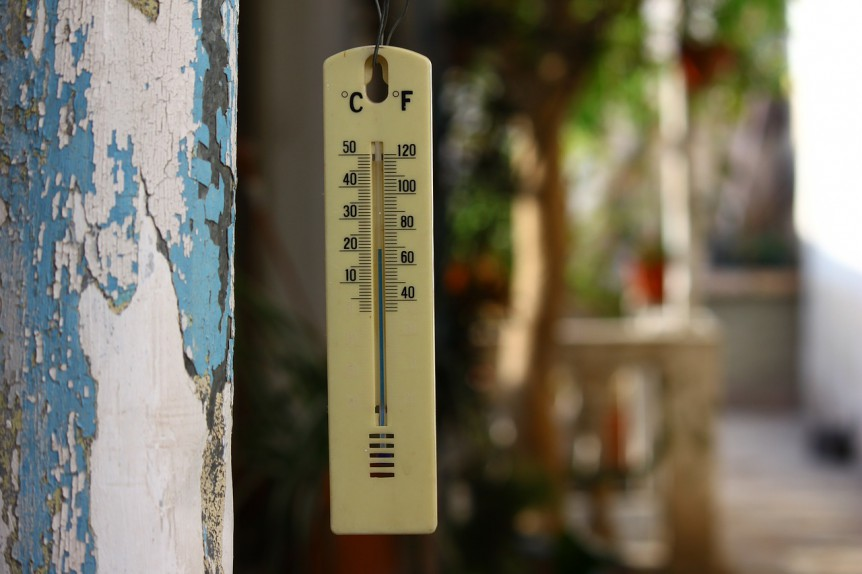# How to convert Fahrenheit to Celsius?

Converting Fahrenheit to Celsius doesn't require a calculator. Here are some easy-to-remember temperatures if you're accustomed to Fahrenheit temperatures...

• 10F is approximately -10C
• 32F = 0C (melting point of water)
• 40F is approximately 4C
• 70F is approximately 20C
• 100F is approximately 40C

## Converting Fahrenheit to Celsius

1. Take your Fahrenheit temperature, and subtract 32 from it. Let's say the temperature is 50F. Our result is then 50 - 32 = 18.
2. Multiply the result by 5. In our example, we do the multiplication and get 18 x 5 = 90.
3. Divide the result by 9 to get our final Celsius result, 90/9 = 10C.

Fahrenheit to Celsius formula: (F-32) * 5 / 9 = C

## Convert Celsius to Fahrenheit

1. Take your Celsius temperature, and multiply by 9. Let's say the temperature is 25C. Our result is then 25 * 9 = 225.
2. Divide the result by 5. In our example, we do the division and get 225 / 5 = 45.
3. Add 32 to the result to get our final Fahrenheit result, 45 + 32 = 77.

Celsius to Fahrenheit formula: C * 9/5 + 32 = F

## Why is -40F = -40C?

Oddly enough, -40F is not colder than -40C. Yes, they are both really cold, but they are also exactly the same temperature. Let's use the formulas from above and try it out.

Convert -40F to Celsius

1. (F-32) * 5 / 9
2. (-40-32) * 5 / 9
3. -72 * 5 / 9 = -40

Convert -40C to Fahrenheit

1. (C * 9 / 5) + 32
2. (-40 * 9 / 5) + 32
3. -72 + 32 = -40

Exactly the same!

1.1.Thanks for those links, Andrew. Also, the rough approximation of doubling and then adding 30 is fantastic. I’ll be updating the post soon with those details.

2.3.1.1.4.Nonono. You must use pencil and paper to perform this conversion even though it is easier, requires less time rather than do what everyone does- whip out your phone and use any of the 100,s of online convertors. And get the answer in mere ssconds.

1.Post
Author

This site uses Akismet to reduce spam. Learn how your comment data is processed.